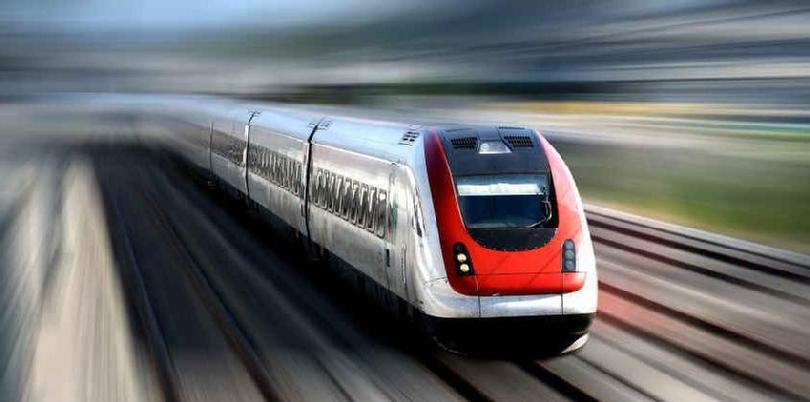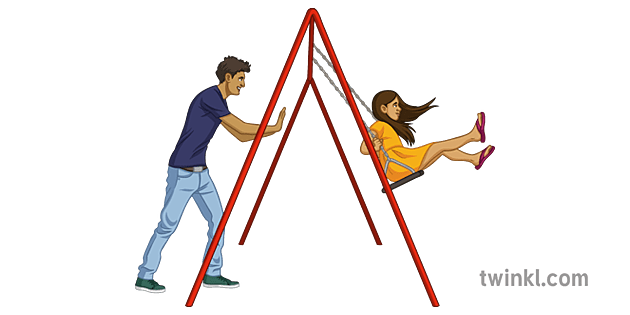Crystal

MS-PS2-2

# Momentum And Impulse Study Guide

In this chapter, we will take a look at how motion can be quantified in terms of momentum and impulse. While the two have the same units, they are different concepts.

# IntroductionSource

You’re out playing with your kid brother and mom on the playground. They’re each on a swing, and it’s your turn to push. You’ll notice that it’s easier to push your kid brother’s swing while it takes a lot more effort and time to get your mom’s swing going. It also takes more effort to bring your mom’s swing to a stop. Common sense tells you that it is because your mom weighs more than your kid brother. But what’s the physics behind it? Impulse and momentum!

## WHAT IS THE MEANING OF MOMENTUM?Source

• We often hear the word momentum used colloquially to describe something gaining movement such that it is difficult to stop.
• We often hear commentators talk about how sports players and political candidates have “gained a lot of momentum”.
• You would experience the same on the playground; it takes more effort to bring a heavier person to stop on a swing. The reason is momentum.
• In physics, momentum is defined as the product of mass and velocity.
• It is a vector quantity and is represented by the lowercase ‘p’.

P = mv

Here, m is the mass of the moving object,

and v is the velocity of the moving object.

The unit for momentum is kg m/s.

In a nutshell, the concept of momentum tells you how much motion there is in a moving object.

## IMPULSESource

• Back in the playground, you will have to apply some amount of force (a push) for a certain amount of time to get the swing moving.
• This is what is called an impulsive force.
• The definition of impulse in physics is force multiplied by the duration for which the force acts.
• Mathematically it is expressed as:

J = F Δt

Here, J is the impulse.

F is the applied force, and

Δt is the duration of time for which the force acts.

• The concept of impulse tells you the effect the applied force has on changing the momentum of an object.
• An impulse can speed up or slow down a moving object.

## RELATION BETWEEN IMPULSE AND MOMENTUM

Consider the impulse and momentum equations:

• Momentum is given mathematically as P = mv. The unit of mass is kilograms (Kg), and the unit of velocity is meters per second m/s. Therefore the unit of momentum is Kg m/s.

• Impulse is defined mathematically as J = F Δt. The unit of force is Newton (N) and the unit of time is seconds (s). Therefore impulse is expressed as Ns.

• However, N can also be expressed as Kg m/. The impulse units then become Kg m/s.

• This equivalence between momentum and impulse is known as the impulse-momentum theorem.

# SUMMARY

• Momentum is defined as the product of mass and velocity. It describes the motion contained in an object.
• Impulse is defined by force multiplied by time. It gives a measure of the change in momentum.
• A large impulse gives a large change in momentum.

## FAQs

Q. What is the application of impulse and momentum?

• Airbags in cars use the principle of the impulse to apply a small but opposite force over a relatively long period of time to dissipate the momentum slowly. This reduces injury.
• If you are catching a falling egg, you move your hand in the direction of the egg to slow it down. Abruptly stopping the egg would break it.

Q. How are momentum and impulse related?
The change in momentum is directly proportional to the impulse given to the object.

We hope you enjoyed studying this lesson and learned something cool about Momentum and Impulse! Join our Discord community to get any questions you may have answered and to engage with other students just like you! We promise, it makes studying much more fun!😎

## SOURCES:

1. Momentum and Impulse: https://flexbooks.ck12.org/cbook/ck-12-physics-flexbook-2.0/section/6.1/primary/lesson/momentum-and-impulse-phys/ Accessed 13th April 2022.
2. Momentum Charge and Impulse Connection: https://www.physicsclassroom.com/class/momentum/Lesson-1/Momentum-and-Impulse-Connection Accessed 13th April 2022.
3. What are Momentum and Impulse? https://www.khanacademy.org/science/physics/linear-momentum/momentum-tutorial/a/what-are-momentum-and-impulse Accessed 13th April 2022.